# GED Math : Mode

## Example Questions

← Previous 1 3 4

### Example Question #1 : Mode

How many modes does this data set: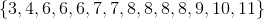NoneExplanation:

The mode of a data set is the element that occurs most frequently. If there is a tie between the frequencies of two (three, etc.) elements, the set has two (three, etc.) modes.

Here, though, only one element (8) appears four times, with no other element appearing more frequently. 8 is the only mode.

### Example Question #2 : Mode

Which of the following elements can be added to the data set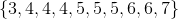to give it exactly one mode?

I:II:III:II and III only

I and III only

Any of I, II, and III

I and II only

I and II only

Explanation:

The mode of the data set is the most frequently occurring element. In the set given, 4 occurs three times, 5 occurs three times, 6 occurs two times, and 3 and 7 occur one time each.

If 4 is added to the data set, then it occurs four times, more than any other element, so it becomes the unique mode; a similar argument holds for 5. If 6 is added to the set, then each of 4, 5, and 6 occur three times, and the set becomes one with three modes.

The correct answer is therefore I and II only.

### Example Question #3 : Mode

Given the data set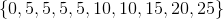, letbe the mean of the set,be the median of the set, andbe the mode of the set. Which of the following is true?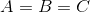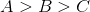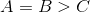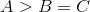Explanation:

The mean of a data set is the sum of its elements divided by the number of elements, which here is 10: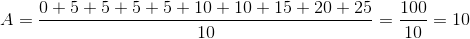The median of a data set with an even number of elements is the mean of the middle two values when the set is arranged in ascending order; the middle numbers are 5 and 10, so the median is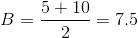The mode of a data set is the most frequently occurring element, which here is 5, so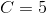.

The correct response is### Example Question #4 : Mode

Veronica went to the mall and bought several small gifts for her sister's birthday. The prices of the items were the following:

$8,$10, $6,$7, $10,$2, $5,$3, $4 What is the mode price of the items? Possible Answers:$6

$2$4.5

$5$10

$10 Explanation: The mode of a set of numbers is the number with the highest frequency (meaning that it shows up the most). Only one number appears twice in this list -$10.

$8,$10, $6,$7, $10,$2, $5,$3, $4$10 is the mode.

### Example Question #5 : Mode

Identify the mode(s) in the set of numbers below: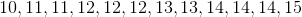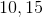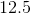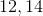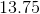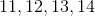Explanation:

The mode(s) of a set of numbers is the number(s) that appear the most frequently. If the numbers in a set only appear once, the set does not have a mode.

In this number set,andappear in the list three times, more often then any other numbers. So,andare the modes.

### Example Question #6 : Mode

Determine the mode for the set of numbers: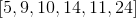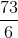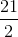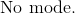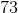Explanation:

The mode is defined as the number or numbers that occur most often in the set of numbers.

Since every number is unique, and only appears once, there is no mode present.

Do not confuse the mode with the median or mean.

The answer is:### Example Question #7 : Mode

Use the following data set of test scores to answer the question: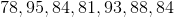Find the mode.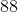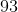Explanation:

To find the mode of a data set, we will find the number that appears most often.

So, given the setwe can see the number that appears most often is 84 (it appears 2 times, while the other numbers only appear once).

Therefore, the mode of the data set is 84.

### Example Question #8 : Mode

A Science class took an exam. Here are the scores of 9 students: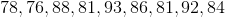Find the mode score.Explanation:

To find the mode score, we will see which score appears most often within the set.

So, given the setwe can see that 81 appears most often (it appears two times, while the others only appear once).

Therefore, the mode score of the data set is 81.

### Example Question #9 : Mode

A class took a Math exam.  Here are the test scores of 10 students.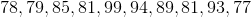Find the mode.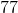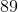Explanation:

To find the mode of a data set, we will find the number that appears most often within the set.

So, given the setwe can see that 81 appears most often (it appears 2 times, while the other scores only appear once). Therefore, the mode of the data set of test scores is 81.

### Example Question #10 : Mode

Identify the mode, if any: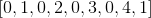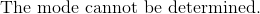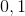Explanation:

The mode includes all numbers that have the highest frequency present.

The only number that appears the most is zero.

Even thoughoccurs twice, it is not the highest frequency.

The answer is:← Previous 1 3 4